Disclaimer: This is an example of a student written essay.

Any opinions, findings, conclusions or recommendations expressed in this material are those of the authors and do not necessarily reflect the views of UKEssays.com.

# Lagrange Multipliers in Mathematics

 ✅ Paper Type: Free Essay ✅ Subject: Mathematics ✅ Wordcount: 1666 words ✅ Published: 8th Sep 2017

Lagrange multipliers arise as a method for maximising (or minimising) a function that is subject to one or more constraints. It was invented by Lagrange as a method of solving problems, in particular a problem about the moons apparent motion relative to the earth. He wrote his work in a paper called Me’chanique analitique (1788) (Bussotti, 2003)

This appendix will only sketch the technique and is based upon information in an appendix of (Barnett, 2009).

Suppose that we have a function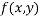which is constrained by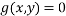. This problem could be solved by rearranging the function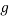for x (or possibly y), and substituting this into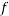. At which point we could then treat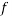as a normal maximisation or minimisation problem to find the maxima and minima.

One of the advantages of this method is that if there are several constraint functions we can deal with them all in the same manner rather than having to do lots or rearrangements.

Considering only f as a function of two variables (and ignoring the constraints) we know that the points where the derivative vanish are: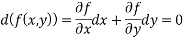Now g can also be minimised and this will allow us to express the equation above in terms of the dx’s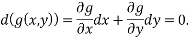Since these are linear functions we can add them to find another solution, and traditionally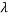is used to getWhich is 0 only when both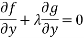We can generalise this easily to any number of variables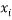and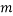constraints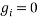as follows: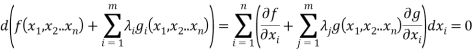We can then solve the various equations for the‘s. The process boils down to finding the extrema of this function: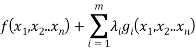As an example imagine that we have a fair 8 sided die. If the die were fair we would expect an average roll of. Let us imagine that in a large number of trials we keep getting an average of 6, we would start to suspect that the die was not fair. We can now estimate the relative probabilities of each outcome from the entropy since we know: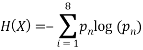We can use Lagrange’s method to solve this equation subject to the constraints that the total probability sums to one and the expected mean (in this case) is 6. The method tells us to minimise the function: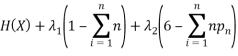Where the first part is the entropy and the other two parts are our constraints on the probability and the mean of the rolls. Differentiating this and setting it equal to 0 we get: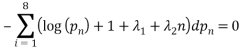Now if we do an integration we know that this value must be a constant function of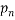since the derivative is 0, also since each of the terms in the summation is 0 we must also have a solution of the form: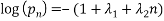OrWe know that the probabilities sum to 1 giving: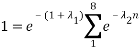Which can be put into (A2.1) to get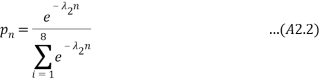Which doesn’t look too much better (perhaps even worse!). We still have one final constraint to use which is the mean value:We can use (A2.2) and re-arrange this to find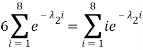Which also doesn’t seem to be an improvement until we realise this is just a polynomial in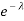: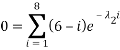If a root,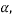exists we can then use it to find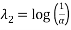. I did not do it that way by hand, I used maple to find the solutionÂ  to the “polynomial”. (the script is below) I also calculated the probabilities for a fair dice as a comparison and test.

 fair dice mu = 4.5 unfair dice mu = 6 p1 0.125 p1 0.32364 p2 0.125 p2 0.04436 p3 0.125 p3 0.06079 p4 0.125 p4 0.08332 p5 0.125 p5 0.11419 p6 0.125 p6 0.15650 p7 0.125 p7 0.21450 p8 0.125 p8 0.29398 lambda = 0 lambda = -0.31521

Table A2. 1: comparison of probabilities for a fair and biased 8sided dice. The bias dice has a mean of 6.

>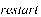>>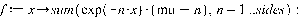>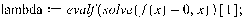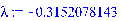>>Equation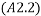also appears in the thermodynamics section.

Becausecan be used to generate the probabilities of the source symbols I think that it would be possible to use this value to characterise the alphabet i.e. take a message from an unknown source and classify the language by finding the closest matchingfrom a list (assuming that the alphabets are the same size). I haven’t done that but think that the same approach as the dice example above would work (the mean would be calculated from the message and we would need more sides!).

When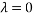we have a totally random source, and in this case the probability of each character is the same. This is easily seen from (A2.2) as all the exponentials contribute a 1 and we are left with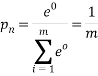Where m is the size of the alphabet – all the symbols are equally probable in this case.

View all

## DMCA / Removal Request

If you are the original writer of this essay and no longer wish to have your work published on UKEssays.com then please:

Related Services

Prices from

S\$203

Approximate costs for:

• 1000 words
• 7 day deliveryHumanity University

Dedicated to your worth and value as a human being!

Related Lectures